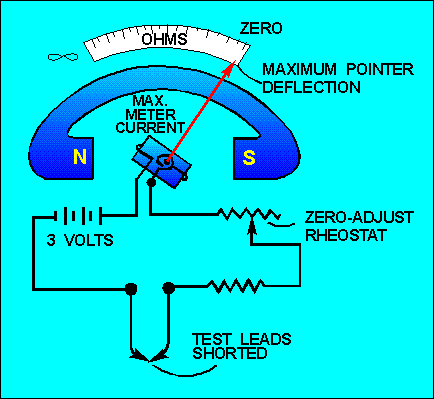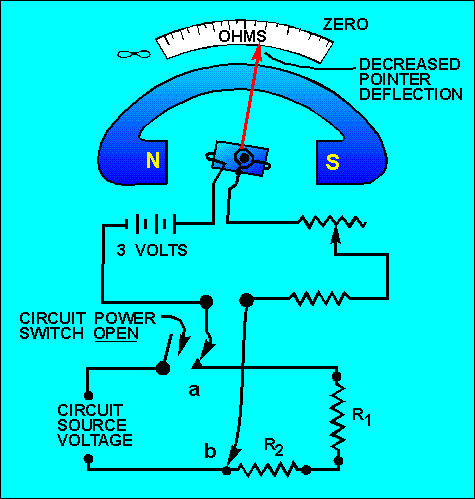Ohmmeters continuity (a complete circuit), or to measure the resistance of a circuit or circuit element, are the OHMMETER and the MEGGER (megohm meter). The ohmmeter is widely used to measure resistance and check the continuity of electrical circuits and devices. Its range usually extends to only a few megohms. The megger is widely used for measuring insulation resistance, such as between a wire and the outer surface of the insulation, and insulation resistance of cables and insulators. ">Custom SearchOHMMETERS The two instruments most commonly used to check the continuity (a complete circuit), or to measure the resistance of a circuit or circuit element, are the OHMMETER and the MEGGER (megohm meter). The ohmmeter is widely used to measure resistance and check the continuity of electrical circuits and devices. Its range usually extends to only a few megohms. The megger is widely used for measuring insulation resistance, such as between a wire and the outer surface of the insulation, and insulation resistance of cables and insulators. The range of a megger may extend to more than 1,000 megohms. The ohmmeter consists of a dc ammeter, with a few added features. The added features are: 1. A dc source of potential (usually a 3-volt battery) 2. One or more resistors (one of which is variable) A simple ohmmeter circuit is shown in figure 1-31. The ohmmeter's pointer deflection is controlled by the amount of battery current passing through the moving coil. Before measuring the resistance of an unknown resistor or electrical circuit, the test leads of the ohmmeter are first shorted together, as shown in figure 1-31. With the leads shorted, the meter is calibrated for proper operation on the selected range. While the leads are shorted, meter current is maximum and the pointer deflects a maximum amount, somewhere near the zero position on the ohms scale. Because of this current through the meter with the leads shorted, it is necessary to remove the test leads when you are finished using the ohmmeter. If the leads were left connected, they could come in contact with each other and discharge the ohmmeter battery. When the variable resistor (rheostat) is adjusted properly, with the leads shorted, the pointer of the meter will come to rest exactly on the zero position. This indicates ZERO RESISTANCE between the test leads, which, in fact, are shorted together. The zero reading of a series-type ohmmeter is on the right-hand side of the scale, where as the zero reading for an ammeter or a voltmeter is generally to the left-hand side of the scale. (There is another type of ohmmeter which is discussed a little later on in this chapter.) When the test leads of an ohmmeter are separated, the pointer of the meter will return to the left side of the scale. The interruption of current and the spring tension act on the movable coil assembly, moving the pointer to the left side (∞) of the scale. Figure 1-31. - A simple ohmmeter circuit.USING THE OHMMETER After the ohmmeter is adjusted for zero reading, it is ready to be connected in a circuit to measure resistance. A typical circuit and ohmmeter arrangement is shown in figure 1-32. Figure 1-32. - Measuring circuit resistance with an ohmmeter.The power switch of the circuit to be measured should always be in the OFF position. This prevents the source voltage of the circuit from being applied across the meter, which could cause damage to the meter movement. The test leads of the ohmmeter are connected in series with the circuit to be measured (fig. 1-32). This causes the current produced by the 3-volt battery of the meter to flow through the circuit being tested. Assume that the meter test leads are connected at points a and b of figure 1-32. The amount of current that flows through the meter coil will depend on the total resistance of resistors R1 and R2, and the resistance of the meter. Since the meter has been preadjusted (zeroed), the amount of coil movement now depends solely on the resistance of R1and R2. The inclusion of R1 and R2 raises the total series resistance, decreasing the current, and thus decreasing the pointer deflection. The pointer will now come to rest at a scale figure indicating the combined resistance of R1 and R2. If R1 or R2, or both, were replaced with a resistor(s) having a larger value, the current flow in the moving coil of the meter would be decreased further. The deflection would also be further decreased, and the scale indication would read a still higher circuit resistance. Movement of the moving coil is proportional to the amount of current flow.Integrated Publishing, Inc. - A (SDVOSB) Service Disabled Veteran Owned Small Business